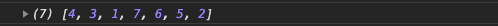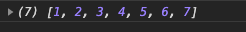• 今天在写代码的时候需要用到一个生成指定范围随机数的函数，百度了一下，发现了很多种方法，这里简单为大家整理一下，方便需要的朋友
• 主要介绍了JavaScript生成指定范围随机数和随机序列,非常不错，具有参考借鉴价值，需要的朋友可以参考下
• 主要介绍了MySQL的指定范围随机数函数rand()的使用技巧,需要的朋友可以参考下
• java 获取指定范围随机数 public Double nextDouble(double min, double max) throws Exception { if (max < min) { throw new BusinessException(CodeMsg.create(40001,"min > max")); ...
java  获取指定范围随机数
public  Double nextDouble(double min,  double max) throws Exception {
if (max < min) {
}
if (min == max) {
return min;
}
Double v = min + ((max - min) * new Random().nextDouble());
String format = String.format("%.5f", v);
return Double.parseDouble(format);
}

展开全文• 数组随机打乱、数组去重、数组并集、数组交集、指定范围随机数
1. 数组随机打乱
function shuffle (arr) {
var result = [],
random;
while (arr.length > 0) {
random = Math.floor(Math.random() * arr.length);
result.push(arr[random])
arr.splice(random, 1)
}
return result;
}
let num = [1,2,3,4,5,6,7];
console.log(shuffle(num));

每次都不一样的2.数组去重利用 set数据类型的特性
function unique(arr) {
if (Array.hasOwnProperty('from')) {
return Array.from(new Set(arr));
} else {
// 兼容不支持set的情况
var n = {},
r = [];
for (var i = 0; i < arr.length; i++) {
if (!n[arr[i]]) {
n[arr[i]] = true;
r.push(arr[i]);
}
}
return r;
}
}
let numTest = [1, 2, 3, 4, 5, 6, 7, 2, 3, 4, 3, 2, 3];
console.log(unique(numTest))

结果3. 交集并集
function unique(arr) {
if (Array.hasOwnProperty('from')) {
return Array.from(new Set(arr));
} else {
var n = {},
r = [];
for (var i = 0; i < arr.length; i++) {
if (!n[arr[i]]) {
n[arr[i]] = true;
r.push(arr[i]);
}
}
return r;
}
}

function union(a, b) {
// 并集
var newArr = a.concat(b);
return unique(newArr);
}

function has(arr, val) {
// 某数组中是否含有某个值
return arr.indexOf(val) != -1 ? true : false;
}

function intersect(a, b) {
// 交集
let num = []
a = unique(a); // 取唯一
for (let o of a) {
if (has(b, o)) {
num.push(o);
}
}
return num;
}
let numA = [1, 2, 3, 4, 5, 3, 5];
let numB = [1, 2, 6, 7, 8, 9, 5];
console.log(union(numA, numB)); // [1, 2, 3, 4, 5, 6, 7, 8, 9]
console.log(intersect(numA, numB)); // [1, 2, 5]

4.  指定范围随机数
function random(min, max) {
return Math.floor(min + Math.random() * ((max + 1) - min))
}
console.log(random(1,9))  // 1-9中的数字



展开全文数组随机打乱 数组去重 数组并集 数组交集
• shell 生成指定范围随机数与随机字符串


shell 生成指定范围随机数与随机字符串

1.使用系统的 $RANDOM 变量 fdipzone@ubuntu:~$ echo $RANDOM17617$RANDOM 的范围是 [0, 32767]

如需要生成超过32767的随机数，可以用以下方法实现。
例：生成400000~500000的随机数

#!/bin/bashfunction rand(){    min=$1 max=$(($2-$min+1))    num=$(($RANDOM+1000000000)) #增加一个10位的数再求余    echo $(($num%$max+$min))}rnd=$(rand 400000 500000)echo$rndexit 0

2.使用date +%s%N
例：生成1~50的随机数

#!/bin/bashfunction rand(){    min=$1 max=$(($2-$min+1))    num=$(date +%s%N) echo$(($num%$max+$min))}rnd=$(rand 1 50)echo $rndexit 0 3.使用/dev/random 和 /dev/urandom /dev/random 存储着系统当前运行环境的实时数据，是阻塞的随机数发生器，读取有时需要等待。 /dev/urandom 非阻塞随机数发生器，读取操作不会产生阻塞。 例：使用/dev/urandom生成100~500的随机数，使用urandom避免阻塞。 #!/bin/bashfunction rand(){ min=$1    max=$(($2-$min+1)) num=$(cat /dev/urandom | head -n 10 | cksum | awk -F ' ' '{print $1}') echo$(($num%$max+$min))}rnd=$(rand 100 500)echo $rndexit 0 4.使用linux uuid uuid 全称是通用唯一识别码，格式包含32个16进制数字，以'-'连接号分为5段。形式为8-4-4-4-12 的32个字符。 fdipzone@ubuntu:~/shell$ cat /proc/sys/kernel/random/uuidfd496199-372a-403e-8ec9-bf4c52cbd9cd
例：使用linux uuid 生成100~500随机数

#!/bin/bashfunction rand(){    min=$1 max=$(($2-$min+1))    num=$(cat /proc/sys/kernel/random/uuid | cksum | awk -F ' ' '{print$1}')    echo $(($num%$max+$min))}rnd=$(rand 100 500)echo$rndexit 0

5.生成随机字符串

例：生成10位随机字符串

#使用date 生成随机字符串date +%s%N | md5sum | head -c 10#使用 /dev/urandom 生成随机字符串cat /dev/urandom | head -n 10 | md5sum | head -c 10

再分享一下我老师大神的人工智能教程吧。零基础！通俗易懂！风趣幽默！还带黄段子！希望你也加入到我们人工智能的队伍中来！https://blog.csdn.net/jiangjunshow
展开全文• shell 生成指定范围随机数与随机字符串 1.使用系统的 $RANDOM 变量 fdipzone@ubuntu:~$ echo $RANDOM17617$RANDOM 的范围是 [0, 32767] 如需要生成超过32767的随机数，可以用以下方法实现。 例：生成400000~500000...
shell 生成指定范围随机数与随机字符串
1.使用系统的 $RANDOM 变量 fdipzone@ubuntu:~$ echo $RANDOM17617$RANDOM 的范围是 [0, 32767]

如需要生成超过32767的随机数，可以用以下方法实现。 例：生成400000~500000的随机数
#!/bin/bash
function rand(){
min=$1 max=$(($2-$min+1))
num=$(($RANDOM+1000000000)) #增加一个10位的数再求余
echo $(($num%$max+$min))
}

rnd=$(rand 400000 500000) echo$rnd

exit 0


2.使用date +%s%N
例：生成1~50的随机数
#!/bin/bash
function rand(){
min=$1 max=$(($2-$min+1))
num=$(date +%s%N) echo$(($num%$max+$min)) } rnd=$(rand 1 50)
echo $rnd exit 0  3.使用/dev/random 和 /dev/urandom /dev/random 存储着系统当前运行环境的实时数据，是阻塞的随机数发生器，读取有时需要等待。 /dev/urandom 非阻塞随机数发生器，读取操作不会产生阻塞。 例：使用/dev/urandom生成100~500的随机数，使用urandom避免阻塞。 #!/bin/bash function rand(){ min=$1
max=$(($2-$min+1)) num=$(cat /dev/urandom | head -n 10 | cksum | awk -F ' ' '{print $1}') echo$(($num%$max+$min)) } rnd=$(rand 100 500)
echo $rnd exit 0  4.使用linux uuid uuid 全称是通用唯一识别码，格式包含32个16进制数字，以’-'连接号分为5段。形式为8-4-4-4-12 的32个字符。 fdipzone@ubuntu:~/shell$ cat /proc/sys/kernel/random/uuid
fd496199-372a-403e-8ec9-bf4c52cbd9cd

例：使用linux uuid 生成100~500随机数
#!/bin/bash
function rand(){
min=$1 max=$(($2-$min+1))
num=$(cat /proc/sys/kernel/random/uuid | cksum | awk -F ' ' '{print$1}')
echo $(($num%$max+$min))
}

rnd=$(rand 100 500) echo$rnd

exit 0


5.生成随机字符串
例：生成10位随机字符串
#使用date 生成随机字符串
date +%s%N | md5sum | head -c 10

#使用 /dev/urandom 生成随机字符串
cat /dev/urandom | head -n 10 | md5sum | head -c 10


展开全文• ## c 生成指定范围随机数

万次阅读 多人点赞 2018-03-23 18:42:28
不过，由于rand()的内部实现是用线性同余法做的，所以生成的并不是真正的随机数，而是在一定范围内可看为随机的伪随机数。 rand： rand()会返回一个0至RAND_MAX之间的随机数值，RAND_MAX的值与int位数有关，最小...
• 生成指定范围内的随机数是一个常用的操作 首先弄明白Math.random()函数，这个函数默认会返回一个大于等于0但小于1的值[0,1) 如果想要生成0~X之间的随机数(不包括X)，只需要将Math.random()返回值乘上X，那么范围...
• 获取指定范围随机数-Math.random（） 公式：Math.random()*(n+1-m)+m，旨在返回[m,n]之间的随机数。 以下为两个实例应用。 获取2至20间的随机100个整数。 @Test public void test3(){ for(int i = 0; i ...Java
• java中获取指定范围随机数的方法： int number = (int)(Math.random()*(maxNumber-minNumber+1)+minNumber); maxNumber:指定范围的最大值 minNumber：指定范围的最小值 举个例子：获取3到10之间的随机数 max...java
• #include <ctime> int ToolRandInt(int min, int max) { srand((unsigned)time(NULL)); int r = rand() % (max - min + 1) + min; return r; }C++
• //随机生成指定范围内的数 function getRandom(min, max) { return Math.floor(Math.random() * (max - min + 1)) + min; }javascriptJAVA
• 生成随机数 生成0-3的随机数 SELECT RAND() * 3 最大不会超过3， SELECT FLOOR(RAND() * 3) 上面生成整数的值是0，1，2，3生成的随机整数是1，2，3的话，语句如下 SELECT FLOOR(1 + RAND()*3) ...
• FLOOR(start_num + RAND() * (end_num - start_num + 1));
• * 根据指定的最小值，和最大值，生成一个随机整数 如参数为 0,100 可以取到0-99之间任意整数 * @param {*} min * @param {*} max 取不到最大值 */ function getRandom(min, max) { return Math.floor(Math....
• mysql rand，round，floor，ceiling函数以及获得指定范围随机数原创 2017年06月11日 16:44:41标签：随机数 /mysql /rand1.rand（）函数只能生成0到1之间的随机小数，如果想要生成0到10,0到100就rand（）*相应的值。...
• 方法一：利用java.util.Random类实现 例如：生成[0,100]内随机数 ...想要得到某个范围内的随机数： （数据类型)(最小值+Math.random()*(最大值-最小值+1)) 例如：生成[0,100]内随机数 int r = (int) (1+M.
• 转载于:https://www.cnblogs.com/dpl9963/p/6115346.html
• 1、生成指定范围随机数：function random(){  return Math.floor(2000 + Math.random()*3000); } //【Math.floor( min + Math.random()*(max - min));】 2、result标识——是否已经生成随机数（生成=需要周期...js...